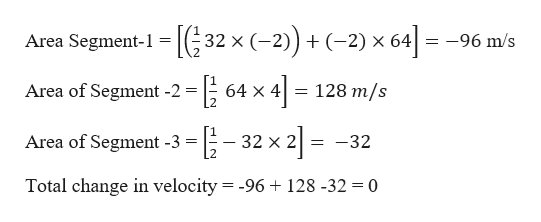# a (m/s2)t (s)62-20

Question
129 views

The figure gives the acceleration a versus time t for a particle moving along an x axis. The a-axis scale is set by as = 16.0 m/s2. At t = -2.0 s, the particle's velocity is 10.0 m/s. What is its velocity at t = 6.0 s?

check_circle

Step 1

Given information:

Scale a-axis = 16 m/s2.

Velocity at t = -2 s is 10 m/s

Velocity at t = 6 s is?

The change in velocity in a-t graph is given by area under the a-t graph

Step 2

Calculation of area a-t graph

Divide the given graph into 3 segments

From -2 to 0 sec segment-1

From 0 to 4 sec segment -2

From 4 to 6 sec ...help_outlineImage Transcriptionclose-[E ))(-2) x 64 Area Segment-1 32 x (2 -96 m/s Area of Segment -2 128 m/s 64 X 4 Area of Segment -3 32 x 2 -32 Total change in velocity = -96 +128 -32 = 0 fullscreen

### Want to see the full answer?

See Solution

#### Want to see this answer and more?

Solutions are written by subject experts who are available 24/7. Questions are typically answered within 1 hour.*

See Solution
*Response times may vary by subject and question.
Tagged in

### Physics